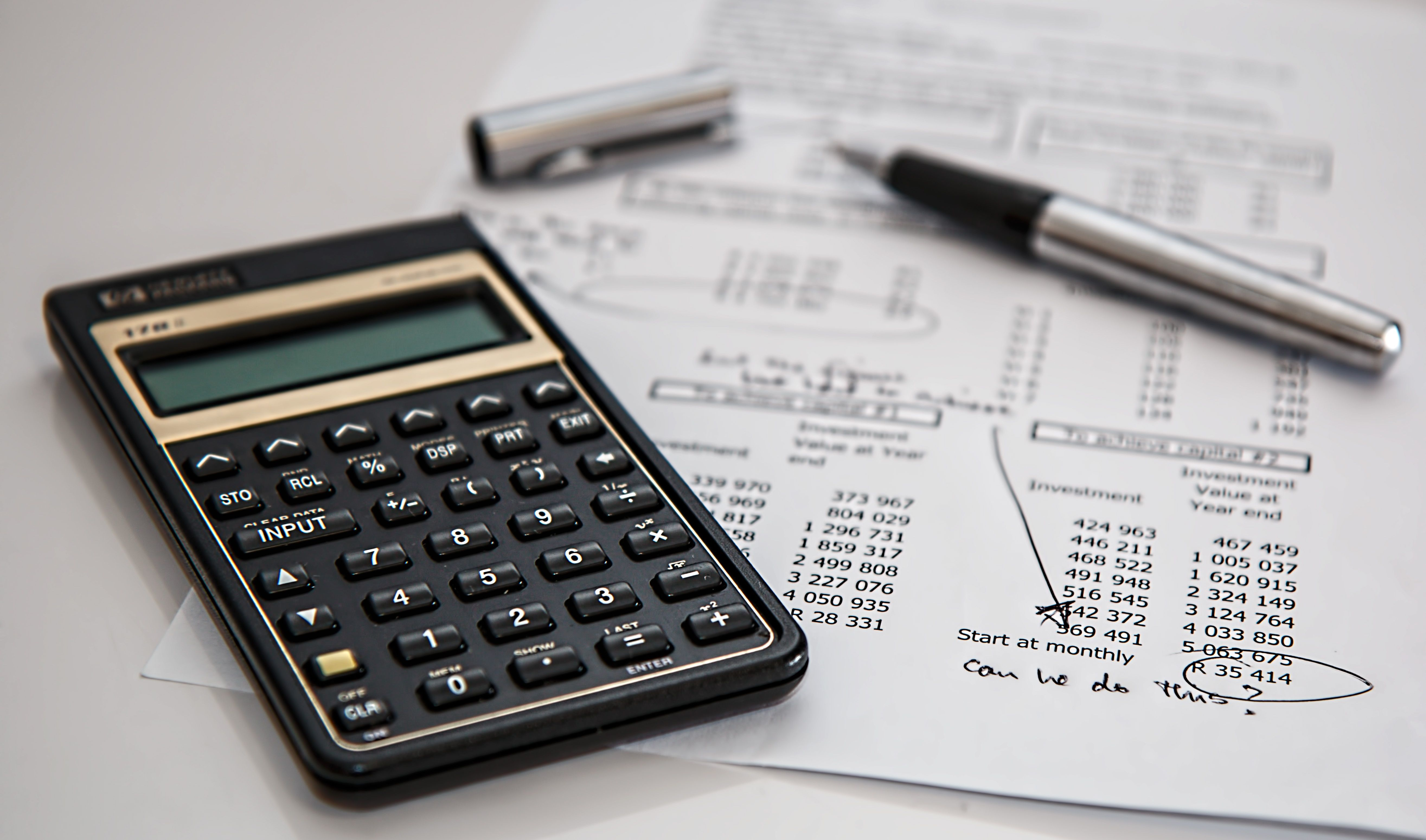# What is applied math useful for?

What is Applied Mathematics Useful For?

# What is Applied Mathematics Useful For?## Introduction

Applied mathematics is the branch of mathematics that involves the application of mathematical principles to real-world problems. It is a highly practical area of study, and its methods and techniques are used in a plethora of different fields, including science, engineering, finance, and many more. In this article, we will explore the different applications of applied mathematics and how they are useful for solving problems in various domains.

## FAQs

### 1. What is applied mathematics?

Applied mathematics consists of the application of mathematical concepts and techniques to solve practical problems in different fields. This field includes the development of new mathematical models and computational tools to solve specific problems, as well as the use of existing techniques to analyze and optimize existing systems or processes.

### 2. What are the different applications of applied mathematics?

Applied mathematics can be applied in various fields such as:

Field of Application Examples
Science Physics, chemistry, biology, environmental science
Engineering Mechanical, civil, electrical, aerospace engineering
Finance Insurance, risk assessment, portfolio optimization
Computer science Artificial intelligence, machine learning, cryptography
Social sciences Economics, political science, psychology

### 3. How is applied mathematics useful for science?

Applied mathematics is essential for scientific research as it provides a set of tools and techniques for modeling and analyzing complex systems. Many scientific problems require mathematical models to accurately represent the underlying phenomena, and applied mathematics provides the necessary frameworks to develop and analyze these models. For example, mathematical models have been used to study weather forecasting, the spread of diseases, and population dynamics in ecology.

### 4. How is applied mathematics useful for engineering?

Engineering problems require mathematical modeling and optimization techniques to design and develop new products and systems. Mathematical models are essential for analyzing and optimizing the performance of existing systems or processes, as well as for predicting the behavior of new systems. For example, applied mathematics is used in mechanical engineering to design aircraft and cars, in civil engineering to design bridges and buildings, and in electrical engineering to design electrical circuits.

### 5. How is applied mathematics useful for finance?

Applied mathematics has a wide range of applications in finance, including portfolio optimization, risk assessment, and option pricing. Financial models require sophisticated mathematical tools to represent the complex interactions between different variables, and applied mathematics provides the necessary techniques to develop and analyze these models. For example, option pricing models are based on mathematical formulas such as the Black-Scholes equation, while portfolio optimization models use techniques such as linear programming and dynamic programming.

## Applications of Applied Mathematics

Applied mathematics has countless applications in various fields, some of which are:

### 1. Robotics

Applied mathematics is used extensively in robotics to design and control robotic systems. Robotic systems require mathematical models to accurately represent their physical interactions with the environment, and applied mathematics provides the tools to develop these models. Additionally, optimization techniques are used to design robotic systems that can perform complex tasks autonomously.### 2. Data Science

Data science is a discipline that involves the use of mathematical and computational techniques to analyze large datasets. Applied mathematics provides the necessary mathematical tools for data analysis and modeling, while computational techniques are used to handle and process large amounts of data efficiently. Many modern data analysis techniques, such as machine learning and neural networks, are based on applied mathematics.### 3. Cryptography

Cryptography is the study of algorithms and mathematical techniques used to secure communication channels and protect data privacy. Cryptographic techniques are based on advanced mathematical concepts, such as number theory and group theory. Applied mathematics provides the necessary theoretical frameworks to develop and analyze these algorithms.## Conclusion

Applied mathematics is a highly practical area of study with countless applications in various fields. It provides the tools and techniques necessary to solve complex problems and optimize existing systems. From robotics to finance, applied mathematics is used in a wide variety of domains, and its importance is only expected to grow in the future.

References: